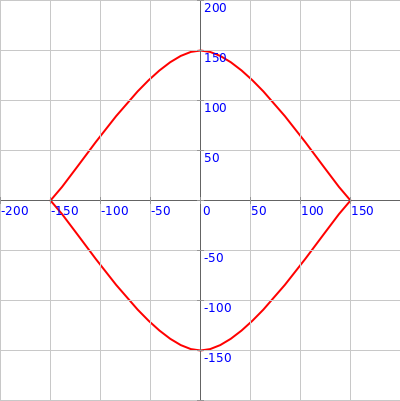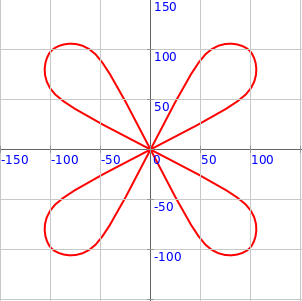## Drawing Vertex based shapes using (r, theta) coordinates

This activity has the following desired goals:

• Learning to draw shapes using vertices at (r, theta) cordinates (A, M).
• Learning to draw curves using vertices at (r, theta) cordinates (A, M).
• Using the above ideas to make interesting curved figures (M, T).

### Step 1

Type in the following code and run it:

``````clear()
showAxes()
showGrid()

def diagonal(x: Double, y: Double) = {
math.sqrt(x * x + y * y)
}

val r1 = diagonal(50, 50)
val r2 = diagonal(100, 50)
val angle2 = math.atan2(50, 100).toDegrees
val r3 = diagonal(100, 100)

beginShape()
vertexRt(r1, 45)
vertexRt(r2, angle2)
vertexRt(r3, 45)
endShape()
``````

Q1a. How does the above code differ from the `Step 1` code in the previous lesson?

Q1b. What does the `vertexRt(r, theta)` command do?

### Step 2

Type in the following code and run it:

``````clear()
setSpeed(fast)
showAxes()
showGrid()

beginShape()
curveVertexRt(150, 0)
curveVertexRt(150, 0)
curveVertexRt(150, 90)
curveVertexRt(150, 180)
curveVertexRt(150, 180)
endShape()
invisible()
``````

Q2a. Read through the code above and try to understand what it does. What does the above code do? How does it do it?

Q2b. What does the `curveVertexRt(r, theta)` command do?

Q2c. How many `curveVertexRt(r, theta)` calls are required to make a curve?

Q2d. Do the first and last `curveVertexRt(r, theta)` calls play a special role in defining the curve to be made?

### Explanation

Command Descriptions:

• `beginShape()` - begins a shape made out of vertices.
• `vertexRt(r, theta)` - adds a vertex to the current shape at the given `(r, theta)` location.
• `curveVertexRt(r, theta)` - adds a curve vertex to the current shape at the given `(r, theta)` location.
• `endShape()` - finishes the current shape and draws it out.

### Exercise

1 Write a program to make the following figure using two different (`beginShape` plus `curveVertexRt`s plus `endShape`) shapes:2 Write a program to make the following figure using four different (`beginShape` plus `curveVertexRt`s plus `endShape`) shapes: